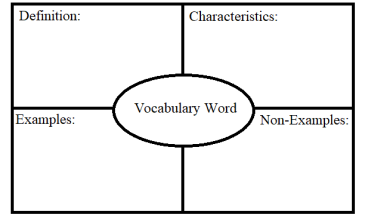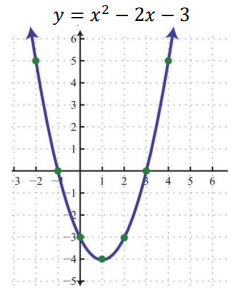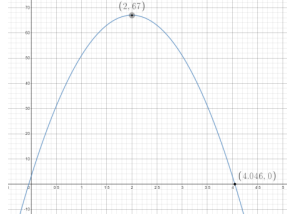# MA.912.AR.3.7Export Print
Given a table, equation or written description of a quadratic function, graph that function, and determine and interpret its key features.

### Clarifications

Clarification 1: Key features are limited to domain; range; intercepts; intervals where the function is increasing, decreasing, positive or negative; end behavior; vertex; and symmetry.

Clarification 2: Instruction includes the use of standard form, factored form and vertex form, and sketching a graph using the zeros and vertex.

Clarification 3: Instruction includes representing the domain and range with inequality notation, interval notation or set-builder notation.

Clarification 4: Within the Algebra 1 course, notations for domain and range are limited to inequality and set-builder.

General Information
Subject Area: Mathematics (B.E.S.T.)
Strand: Algebraic Reasoning
Status: State Board Approved

## Benchmark Instructional Guide

### Terms from the K-12 Glossary

• Coordinate Plane
• Domain
• Function Notation
• Range
• $x$-intercept
• $y$-intercept

### Vertical Alignment

Previous Benchmarks

Next Benchmarks

### Purpose and Instructional Strategies

In grade 8, students graphed linear two-variable equations given a table, written description or equation. In Algebra I, students graph quadratic functions given this same kind of information. In later courses, this work expands to other families of functions.
• Instruction includes conversations about interpreting $y$-intercepts; intervals where the function is increasing, decreasing, positive or negative; end behavior; and symmetry.
• When discussing end behavior, students should see a relationship between the sign of '$a$' and the end behavior the function exhibits. Instruction presents students with the equation of a function first, before showing its graph and asking them to predict its end behavior (MTR.5.1).
• Depending on the form the function is presented in, students may be able to predict other features as well (MTR.5.1).
• Instruction includes making connections to various forms of quadratic equations to show their equivalency. Students should understand and interpret when one form might be more useful than other depending on the context.
• Standard Form Can be described by the equation $y$ = $a$$x$2  + $b$$x$ + $c$, where $a$, $b$ and $c$ are any rational number. This form can be useful when identifying the $y$-intercept.
• Factored form Can be described by the equation $a$($x$$r$1) ($x$$r$2), where $r$1 and $r$2 are real numbers and the roots, or $x$-intercepts. This form can be useful when identifying the $x$-intercepts, or roots.
• Vertex form Can be described by the equation $y$ =$a$($x$$h$)2 + $k$, where the point ($h$, $k$) is the vertex. This form can be useful when identifying the vertex.
• Instruction includes the use of $x$-$y$ notation and function notation.
• Instruction includes representing domain and range using words, inequality notation and set-builder notation.
• Words If the domain is all real numbers, it can be written as “all real numbers” or “any value of $x$, such that $x$ is a real number.”
• Inequality notation If the domain is all values of $x$ greater than 2, it can be represented as $x$ > 2.
• Set-builder notation If the domain is all values of $x$ less than or equal to zero, it can be represented as {$x$|$x$ ≤ 0} and is read as “all values of $x$ such that $x$ is less than or equal to zero.”
• Instruction includes the use of appropriately scaled coordinate planes, including the use of breaks in the $x$- or $y$-axis when necessary.

### Common Misconceptions or Errors

• When describing intervals where functions are increasing, decreasing, positive or negative, students may represent their interval using the incorrect variable. In these cases, ask reflective questions to help students examine the meaning of the domain and range in the problem.
• Students may miss the need for compound inequalities in their intervals. In these cases, refer to the graph of the function to help them discover areas in their interval that would not make sense in context.

### Strategies to Support Tiered Instruction

• Teacher provides the opportunity to complete a graphic organizer to compare related key features.
• For example, students can compare domain versus range, increasing versus decreasing or positive versus negative.
• Instruction includes modeling how to sketch the graph of the function to determine where the graph is increasing or decreasing. Students must understand that graphs are read left to right.
• Instruction includes reflective questions to examine the meaning of the domain and range in the problem.
• For example, students can ask why the range does not contain all real numbers if the domain does contain all real numbers.
• For example, students can ask how the vertex relates to the domain and range.
• Instruction references the graph of the function to help discover areas in compound inequality intervals that would not make sense in context.
• Teacher provides a graphic organizer for key features (vertex; symmetry; end behavior; intercepts) which can be completed using information provided in a given problem.• Teacher provides a picture of a parabola (ensure the vertex and zeros are visible) and labels the parabola with a few of the key features. Then, teacher instructs students to label the parabola with the remaining key features.
• For example, label the parabola with the coordinates of the vertex, axis of symmetry, minimum or maximum and zeros.• A punter kicks a football to the opposing team. The trajectory of the football can be modeled by the function $h$($t$) = −16$t$2+ 64$t$ + 3 where $h$($t$) represents the height of the football at any point in time (in seconds), $t$. A graph of the function is below.• Part A. Determine the meaning of the $y$-intercept in this context.
• Part B. How high did the punt go?
• Part C. Over what interval was the football increasing in height? When was the height decreasing?
• Part D. What domain and range of this function is appropriate for the context?

• A company plans to build a large multiplex theater. The financial analyst told her manager that the profit function for their theater was $P$($x$) = −$x$2+ 48$x$ −512, where $x$ is the number of movie screens, and $P$($x$) is the profit earned in thousands of dollars.
• Part A. Determine the range of production of movie screens that will guarantee that the company will not lose money.
• Part B. What is the optimal number of movie screens the theater should have?

### Instructional Items

Instructional Item 1
• Graph the function $f$($x$) = $x$2+ 2$x$ − 3. Identify the domain, range, vertex and zeros of the function.

*The strategies, tasks and items included in the B1G-M are examples and should not be considered comprehensive.

## Related Courses

This benchmark is part of these courses.
1200310: Algebra 1 (Specifically in versions: 2014 - 2015, 2015 - 2022, 2022 and beyond (current))
1200320: Algebra 1 Honors (Specifically in versions: 2014 - 2015, 2015 - 2022, 2022 and beyond (current))
1200380: Algebra 1-B (Specifically in versions: 2014 - 2015, 2015 - 2022, 2022 and beyond (current))
1200400: Foundational Skills in Mathematics 9-12 (Specifically in versions: 2014 - 2015, 2015 - 2022, 2022 and beyond (current))
7912090: Access Algebra 1B (Specifically in versions: 2014 - 2015, 2015 - 2018, 2018 - 2019, 2019 - 2022, 2022 and beyond (current))
1200315: Algebra 1 for Credit Recovery (Specifically in versions: 2014 - 2015, 2015 - 2022, 2022 and beyond (current))
1200385: Algebra 1-B for Credit Recovery (Specifically in versions: 2014 - 2015, 2015 - 2022, 2022 and beyond (current))
7912075: Access Algebra 1 (Specifically in versions: 2014 - 2015, 2015 - 2018, 2018 - 2019, 2019 - 2022, 2022 and beyond (current))
1200710: Mathematics for College Algebra (Specifically in versions: 2022 and beyond (current))
1209315: Mathematics for ACT and SAT (Specifically in versions: 2022 and beyond (current))

## Related Access Points

Alternate version of this benchmark for students with significant cognitive disabilities.
MA.912.AR.3.AP.7: Given a table, equation or written description of a quadratic function, select the graph that represents the function.

## Related Resources

Vetted resources educators can use to teach the concepts and skills in this benchmark.

## Formative Assessment

Type: Formative Assessment

## Lesson Plans

Parts and more Parts-- Parabola Fun:

This is an entry lesson into quadratic functions and their shapes. Students see some real-life representations of parabolas. This lesson provides important vocabulary associated with quadratic functions and their graphs in an interactive manner. Students create a foldable and complete a worksheet using their foldable notes.

Type: Lesson Plan

This lesson covers quadratic translations as they relate to vertex form of a quadratic equation. Students will predict what will happen to the graph of a quadratic function when more than one constant is in a quadratic equation. Then, the students will graph quadratic equations in vertex form using their knowledge of the translations of a quadratic function, as well as describe the translations that occur. Students will also identify the parent function of any quadratic function as $f\left(x\right)={x}^{2}$.

Type: Lesson Plan

## Perspectives Video: Expert

Using Mathematics to Optimize Wing Design:

Nick Moore discusses his research behind optimizing wing design using inspiration from animals and how they swim and fly.

Type: Perspectives Video: Expert

## Tutorials

Graphing Quadractic Functions in Vertex Form:

This tutorial will help the students to identify the vertex of a parabola from the equation, and then graph the parabola.

Type: Tutorial

This tutorial helps the learners to graph the equation of a quadratic function using the coordinates of the vertex of a parabola and its x- intercepts.

Type: Tutorial

## Student Resources

Vetted resources students can use to learn the concepts and skills in this benchmark.

## Tutorials

Graphing Quadractic Functions in Vertex Form:

This tutorial will help the students to identify the vertex of a parabola from the equation, and then graph the parabola.

Type: Tutorial